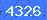# 音箱分频器在线计算公式

↓本文不许可它人转载,只许可引用链接.

 C1= μf L1= mH. 相移的一阶分频器是90度

 C1= μf L1= mH C2= μf L2= mHC1=μf L1=mH C2=μf L2=mH L3=mH C3=μfC1=μf C2=μf L2=mH L1=mH C3=μf L3=mH C4=μf L4=mHC1= μf R1= 欧姆L型扬声器喇叭衰减电路

 R1=欧姆 R2=欧姆发表评论

 验证码:看不清楚,点击刷新

www.838dz.com